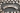# Variable Expressions: Practice Problems

POSTED ON APRIL 15, 2020## Lesson Intro: Expressions with Variables

In this lesson, Juni Mathematics instructor Kadyn talks about variables and variable expressions - foundational concepts in pre-algebra. Variables are important to know for higher levels of math, and are even used similarly in other subjects like computer science!

Read Kadyn's Intro to Variable Expressions lesson first to understand what variables are and how to use them in expressions. Then, use what you've learned with Kadyn's warmup questions on this page to solidify your understanding, and practice solving basic arithmetic problems with different variables.

Once you've checked your answers, you can also keep practicing using variables with Variable Expressions Drills and Word Problems.

## Warmup Problems

### For Problems 1-3, simplify the following expressions.

#### Problem 1:

Practice working with variables for addition and subtraction.

• x + 3x
• x - (-7x)
• (3 + 2x) - (5x - 7)
• 4x + (2 - (-3x + 7) - 5 - 2x)
• -3x - 3 + (2x - (5 + 7x))

#### Problem 2:

Practice working with variables for multiplication and division.

• 6 ⋅ 2y
• 3y ⋅ 4
• 3 ⋅ (3y + 2)
• 5 - |3 - 6|) ⋅ 2y
• (2 + 1) ⋅ 4y ÷ 2

#### Problem 3:

Practice simplifying expressions with multiple variables.

• 2x + 3x - 5y + y
• 3y + 7x - (5y - 2x)
• y + 2x - 3z + 4y - 7x - 6z
• 4 ⋅ (2x + y - 8) - (7x + 3y - 17)
• (3x + 9y + 18) ÷ 3

#### Problem 4:

Evaluate the following expressions with the given value for x.

• 3x
where x = 5
• 7x - 4 ⋅ 5
where x = 3
• 2 - (3 - 4x)
where x = -1
• (5 - (5 ⋅ 3 - 10x)) ÷ 5
where x = 2
• (-4x + 6) - (2 ⋅ (-5x + 4))
where x = -2

#### Problem 5:

Evaluate the following expressions with the given values for x and y.

• 5x - 2y + 7
where x = -1, y = -5
• 3x2 + 3 ⋅ (7 - 2y)
where x = 3, y = 2
• y + (-2 ⋅ (3x - 2y)) + (7 - 2x)
where x = 4, y = -1

## Solutions

### Problem 1:

• 4x
• 8x
• 3 + 2x - 5x + 7 = -3x + 10
• 4x + 2 + 3x - 7 - 5 - 2x = -3x - 10
• -3x - 3 + 2x - 5 - 7x = -8x - 8

### Problem 2:

• 12y
• >12y
• 9y + 6
• (5 - 3) ⋅ 2y = 4y
• 12y ÷ 2 = 6y

### Problem 3:

• 5x - 4y
• 3y + 7x - 5y + 2x = 9x - 2y
• -5x - 6y - 9z
• (8x + 4y - 32) - (7x + 3y - 17) = x + y - 15
• x + 3y + 6

### Problem 4:

• 3 ⋅ 5 = 15
• 7 ⋅ 3 - 4⋅ 5 = 21 - 20 = 1
• 2 - 3 + 4x = -1 + 4 ⋅ -1 = -5
• (5 - 15 + 10x) ÷ 5 = (-10 + 10x) ÷ 5 = -2 + 2x = -2 + 2 ⋅ 2 = 2
• -4x + 6 - (-10x + 8) = -4x + 6 + 10x - 8 = 6x - 2 = 6 ⋅ (-2) - 2 = -14

### Problem 5:

• 5 ⋅ (-1) - 2 ⋅ (-5) + 7 = -5 + 10 + 7 = 12
• 3 ⋅ (3^2) + 3 ⋅ (7 - 2 ⋅ 2) = 3 ⋅ 9 + 3 ⋅ (3) = 27 + 9 = 36
• y + (-6x + 4y) + 7 - 2x = -8x +5y + 7 = -8 ⋅ 4 + 5 ⋅ (-1) + 7 = -32 - 5 + 7 = -30

## More Exercises on Variables

We hope you enjoyed Kadyn's Warmup Problems with Variable Expressions! This lesson falls under our Pre-Algebra A course curriculum. Continue practicing variables with drills and word problems below. Or, review key terms and concepts with Kadyn's Intro to Variable Expressions lesson.

## Need help or want to keep learning?

To keep practicing or learning, please check out all of our math and coding tutorials on our Home Learning Resources page.

Need help? Looking up your questions is one of the best ways to learn! Another great way to learn is from an experienced math instructor. Learn more about our online math courses.